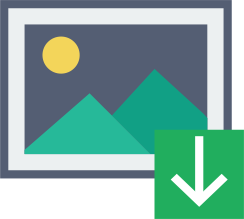## 将军笔记之图形初阶

2017-07-26 coco 生信菜鸟团 生信菜鸟团

1. 在交互式会话中，可以通过逐条输入语句构建图形，逐渐完善图形特征，直到得到想要的结果。

dev.new()

dev.new()

2. 一个大栗子：

plot()函数画图

plot()是R中为对象做图的一个泛型函数，意思就是说，它的输出将根据所绘制对象类型的不同而不同。

type="b"表示同时绘制点和线。

• 使用par()函数通过修改图形参数来定义图形特征（字体，颜色，坐标轴，标题）：

par()函数的返回值

par()函数返回的就是一个列表，内容是图形的各种参数。

• 指定图形参数的第二种方法，为高级绘图函数直接提供选项键值对。plot(does,drugA,type="b",lty=2,pch=17)

• 符号和线条：几个设置符号和线条的参数：

• pch=整数（0-25），指定绘制点时用的符号。对于符号21-25，还可以指定边界颜色（col=）和填充颜色（bg=）。pch是plotting character的缩写；

• cex=浮点数，指定pch规定的符号的大小。数值表示的是符号相对于默认大小的缩放倍数。cex可能是character expansion（字符缩放因子）（这里的character应该指的都是绘图上的点）的缩写

• lty=整数（1-6），指定线条类型；lty是line type的缩写；

• lwd=浮点数，指定线条宽度。数值表示默认宽度的倍数。lwd是line width的缩写；

• 使用实例：plot(dose,drugA,type="b",lty=3,lwd=3,pch=15,cex=2)

• 颜色：

• col：默认的绘图颜色。lines(), pie()可以接受一个含有颜色值的向量，并自动循环使用，比如col=c("red","blue"); 这怎么和pch的边界颜色区分啊？

• col.axis：坐标轴刻度文字的颜色。注意是刻度文字颜色，不是坐标轴颜色，也不是坐标轴名称的颜色；

• col.lab：坐标轴标签颜色（就是坐标轴名字的颜色）；

• col.main：标题颜色；

• col.sub：副标题颜色；

• fg：图形前景色；

• bg：图形背景色。

• 表示颜色的方法：col=1; col="white"; （果然1是white呀！后面有个地方使用颜色从2开始，就是这个原因了）col="#FFFFFF"; col=rgb(1,1,1); col=hsv(0,0,1)都是白色

• colors()函数返回所有颜色的名字，一千多种；

• 创建连续型颜色向量的函数：rainbow(), heat.colors(), terrain.colors(),topo.colors(),cm.colors()。rainbow(10)可以生成10种连续的彩虹型颜色。gray()生成多阶灰度色，用值在0-1之间的向量来指定颜色灰度。（返回的都是字符。是绘图函数把这些字符解释成了颜色）

• 例子：

rep(1,n)函数返回一个向量，向量中包含n个1；

• 文本属性：

• 指定文本大小的参数：

• cex：同前；（奇怪，cex指定的是点的大小啊...）（确实是图点的大小）

• cex.axis：坐标轴刻度文字大小；

• cex.lab：坐标轴标签

• cex.main：标题缩放

• cex.sub：副标题缩放。（怎么没有个cex.legend...）

• 指定字体字号字样的参数（字号不就是字的大小么？？？）

• font=整数：字体样式：1常规，2粗体，3斜体，4粗斜体，5符号字体

• font.axis

• font.lab

• font.main

• font.sub

• ps=：字体磅值，文本最终大小为ps*cex

• family=""：字体族（取值为serif（衬线），sans（无衬线），mono（等宽））、

• 一个例子：par(font.lab=3,cex.lab=1.5, font.main=4,cex.main=2)

• family的取值也可以自己设定：windowsFonts(A=windowsFont("Arial Black"),B=windowsFont("Bookman Old Style"),C=windowsFont("Comic Sans MS"))。注意函数名字是复数，windowsFonts，里面的参数是单数，windowsFont。执行这个函数后，family选项的取值就成了A,B,C.family="A"(注意引号)。注意windowsFont()仅在Windows中有效，在苹果上，要用quartzFonts()

• 对于PDF文件，使用pdf(file="myplot.pdf",family="fontname")生成图形。fontname通过函数names(pdfFonts())函数来找。

• 对于postscript图形，则是names(postscriptFonts())和postscript(file="myplot.ps",family="fontname")。注意引号。

• 图形尺寸和边界尺寸

• pin=c(a,b) 以英寸表示图形的宽和高

• mai=c(a,b,c,d) 以英寸表示边界大小，顺序是下左上右；

• mar=c(a,b,c,d) 以英分（十二分之一英寸）表示边界大小，顺序下左上右；

• 例：par(pin=c(4,3),mai=c(1,.5,1,.2))

• 一个综合例子：

• 输入数据：

• dose <- c(20,30,40,45,60)

• drugA <- c(16,20,27,40,60)

• drugB <- c(15,18,25,31,40)

• 通过par()函数，设定一些图形参数：（那是不是我看看par()里面都有些啥，就知道图形到底有多少选项了？）

• par(pin=c(2,3))

• par(lwd=2,cex=1.5)

• par(cex.axis=.75,font.axis=3)

• 做图，并在plot()函数中也指定了一些图形参数

• plot(dose,drugA,type="b",pch=19,lty=2,col="red")

• plot(dose,drugB,type="b",pch=23,lty=6,col="blue",bg="green")

3. 添加文本、自定义坐标轴和图例

plot(dose,drugA,type="b",

col="red",lty=2,pch=2,lwd=2,

main="Clinical Trials for Durg A",

sub="This is hypothetical data",

xlab="Dosage",ylab="Drug Response",

xlim=c(0,60),ylim=c(0,70))

main="xxx"，设置主标题；

sub="xxx"，设置副标题；

xlab="xxx", ylab="xxx"，设置x轴y轴名称；

xlim=c(a,b),ylim=c(c,d)，设置x轴y轴范围

• 标题

• title()函数：title(main="main title",col.main="red",sub="sub-title",col.sub="blue",xlab="x-axis label",ylab="y-axis label",col.lab="green",cex.lab=.75) 自己揣摩；(title函数不仅可以设置图标题，还可以设置标题颜色；还可以设定副标题及其颜色以及坐标轴标题及其颜色)

• 坐标轴

• 使用axis()时，应该禁用绘图函数自动生成的坐标轴：axes=FALSE禁用全部

• axis(side,at=,labels=,pos=,lty=,col=,las=,tck=)

• side：一个整数，表示在图形的哪边绘制坐标轴，1234=下左上右；

• at=数值，表示需要绘制刻度线的位置

• labels 字符向量，表示刻度线旁边的文字标签；（如果是NULL，则使用at中的值）

• pos，坐标轴线绘制位置的坐标（即与另一条坐标轴相互位置的值）

• lty线条类型

• col线条和刻度颜色

• las标签是否平行于（0）或垂直于（=2）坐标轴（诶？1呢？）

• tck刻度线长度，以相对于绘图区域大小的分数表示，负值表示在图形外，正值表示在图形内，0表示不用刻度，1表示绘制网格线，默认-0.01

• 坐标轴，xaxt=“n”和yaxt=“n”分别禁用x轴和y轴（留下框架线，去除刻度）

• 例子：

par()函数设置边界。+0.1表示5.1，4.1，4.1，8.1么？为什么要这样写？减少输入？？？

yaxt="n"，表示禁用y轴，仅留下框架线，去掉刻度线；ann=FALSE移除默认的标题标签

lines()函数，可以为一幅现有图形添加新的图形元素（和abline()什么区别？）；执行后的图形如下所示：

axis函数中，2的位置对应的就是side，1234分别表示下左上右，2表示在图形的左侧绘制坐标轴；at表示坐标轴上写数字的位置，at=x表示就在x表示的那些位置写标签（这里的变量指的是x那个变量）；标签内容用labels规定，还是x的内容，表示在at规定的位置上写x的内容；las=2表示标签垂直于坐标轴（las是什么的缩写？）。labels=round(z,digits=2)大概是规定内容的同时要规定数字选项的话，要用round函数放在一起？？？2017/04/12 21:53 round函数是四舍五入的意思，所以这里的意思是labels使用z的值，但是要把z四舍五入到两位数。执行完后图形如下所示：

mtext()用于在图形的边界添加文本。使用起来和axis()还是蛮像的，只是前面第一个参数是文本内容。里面的line=3选项意思是调整文本与图形的距离；执行后图形如下所示：

title()函数可以为图和坐标轴都加上标题。

• 次要刻度线

• 创建次要刻度线需要使用Hmisc包中的minor.tick()函数：minor.tick(nx=n,ny=n,tick.ratio=n)

• nx, ny分别指定x轴和y轴每两条主刻度线之间的区间个数；tick.ratio表示次刻度线长度相对主刻度线长度的比例。

• par("tck")可以得到当前主刻度线的长度（要这个值干嘛？这个函数用不上啊？ 2017/04/12 21:53 在minor.tick函数中，tick.ratio参数可能需要知道主刻度线的长度（既然是比例，那不知道也行其实...）

• 参考线

• 函数abline()可以为图形添加参考线：abline(h=yvalues,v=xvalues)（这里都讲过，我后面都忘了...）.

• 例如：abline(h=c(1,5,7))会在y轴1，5，7位置上添加水平线

• abline(v=seq(1,10,2),lty=2,col="blue")会在x轴1，3，5，7，9位置上添加垂直的蓝色虚线。

• 图例：

• 使用legend()函数添加图例：legend(location, title, legend)

• location：指定图例位置：

• 直接给定图例的x, y坐标，

• 执行locator(1),通过鼠标单击给出图例位置

• 使用关键字bottom，bottomleft，left，topleft，top，topright，right，bottomright或center放置图例

• 如果使用了上述关键字，可以同时使用参数inset=指定图例向图形内侧移动的大小

• title：字符串，图例标题

• legend：图例标签组成，字符向量。

• col=, pch=, lwd=, lty=, fill= 用于创建颜色填充的盒形。

• 指定盒子样式bty，指定背景颜色bg，指定大小cex，指定文本颜色text.col，指定horiz=TRUE水平放置图例。

• 例子：

• abline(h=c(30),lwd=1.5,lty=2,col="gray")在y=30处加一条水平线。注意即使只加一条线，也要写在c(30)里面；（前面那句话说错了。试了试，只添加一条线的话，不用非得写在c()里面，直接写就行了）

• library(Hmisc)

• minor.tick(nx=3,ny=3,tick.ratio=.5) 次刻度线把主刻度线分成三份，长度是主刻度线的一半；

• legend("topleft",inset=.05,title="Drug Type",c("A","B"),lty=c(1,2),pch=c(15,17),col=c("red","blue")) 注意title之后，是legend的内容，直接给个向量；（图例和图形内容的对应，全靠手工吗？）

• 文本标注：

• 通过函数text()和mtext()将文本添加到图形上。

• text()可向绘图区域内部添加文本，text(location,"text",pos,)

• mtext()则向图形的四个边界之一添加文本:mtext("text",side,line=n,...)

• location可以是x,y坐标，也可以通过指定location为locator(1)使用鼠标指定位置；

• pos，文本相对于位置参数的方位，1234=下左上右；可同时指定offset=作为偏移量。（location确定所在地点，pos确定在地点的下方左方上方右方）

• text()也可以用来表示图形中的点。示例：

• > plot(wt,mpg,

• + main="Mileage vs. Car Weight",

• + xlab="Weight",ylab="Mileage",

• + pch=18,col="blue") 先画个图，没啥好解释的；

• > text(wt,mpg,

• + row.names(mtcars),

• + cex=0.6,pos=4,col="red") 这里展示的是用text()函数给每个点加标签的功能，加的标签的内容是数据集的rownames；然而前面的wt,mpg怎么理解？（wt,mpg的位置应该是location，location可以是xy坐标，所以这里wt,mpg应该是提供了文本的xy坐标，然后pos指定文本在坐标的右方）

• 又一个例子：

• text(3,3,"Example of default text")

• text(4,4,family="mono","example of monospaced text")

• text(5,5,family="serif","example of serif text")

• 上面例子主要展示使用xy坐标来指定text的位置，以及使用family=“”指定文本字体。

• 数字标注：

• 可以为图形添加数学符号和公式，详见help(plotmath)，书上讲得不详细，我就不记了。

2017/03/19 13:42

4. 图形的组合：

• par(mfrow=c(m,n)) 创建一个m行n列的格子，每个格子放一个图，按行放；

• par(nfcol=c(m,n)) 创建一个m行n列的格子，每个格子放一个图，按列放。

• 例子：

• par(mfrow=c(2,2)) 先画一个2*2的格子，在格子中按行放图；

• plot(wt,mpg,main="Scatterplot of wt vs. mpg") 第一个图，会出现在左上角的格子里；

• plot(wt,disp,main="Scatterplot of wt vs disp") 第二个图，会出现在右上角的格子里；

• hist(wt,main="Histogram of wt") 第三个图，会出现在左下角的格子里；

• boxplot(wt,main="Boxplot of wt") 第四个图，会出现在右下角的格子里。四个图，画一个出现一个。最终结果如下图所示。

• 第二个例子：

• par(mfrow=c(3,1)) 也是规定几个格子，不过这次是3*1的格子，即3行，一列，三个图竖着排一列；

• hist(wt)

• hist(disp)

• hist(mpg) 上面三个图，会竖着排一列。如下图所示。注意hist()函数会自动生成一个默认的图题。

par(mfrow(3,1))的排列

• layout()函数的调用形式为layout(matrix)，其中matrix是一个矩阵，指定了要组合的多个图形所在的位置。

• 例子：

• layout(matrix(c(1,1,2,3),2,2,byrow=TRUE)) 一幅图被置于第一行，另两幅图被置于第二行。但是不知道具体是怎么指示的？书上也没讲。

• 里面的matrix()函数能看懂，意思是建立了一个矩阵，矩阵的数字是1，1，2，3，排成两行两列，按行排列。意思是不是：总共划四个格子，按行排成2*2形式，其中第一行两个值是1，意思是用来放第一个图，左下角值是2，用来放第二个图，右下角值是3，用来放第三个图？如果是这样的话，那如果我写layout(matrix(c(2,3,1,1),nrow=2,ncol=2,byrow=TRUE))，那么就会出现下面是第一幅图，上面分别是第二第三幅图。试试看！果然跟我想得一模一样！代码：layout(matrix(c(2,3,1,1),nrow=2,ncol=2,byrow=TRUE))；hist(wt)；hist(mpg)；hist(disp)；结果：（再解释一遍：生成的矩阵中的数字是指示用来放第几幅图的。比如一个内容为(1,1,2,3)的2*2矩阵，就表示左上右上位置放第一幅图(因为这两个位置的数字是1)，左下角放第二幅图(因为这里的位置是2)，右下角放第三幅图(因为这里的位置是3)）

• 可以在layout()函数中使用widths=c(a,b,c...)和heights=c(a,b,c...)来指定各列的宽度比例和各行的高度比例。

• layout(matrix(c(1,1,2,3),2,2,byrow=TRUE),widths=c(3,1),heights=c(1,2))第一列和第二列的宽度比3：1；上行与下行高度比为1：2。绝对高度需要用lcm()函数指定，单位是厘米。

• 更精细的调控

• 把几个图合并在一个图里面。将整个绘图区域想象成：左下角坐标为（0，0），右上角坐标为（1，1），fig=c()所指定的向量，四个值分别是图的：左下角x坐标，右下角x坐标，左下角y坐标，右上角y坐标。这四个值能确定一个矩形区域，就是下面要画的图所在的区域。

• 代码示例：

• par(fig=c(0,0.8,0,0.8)) 指定一个矩形区域

• plot(wt,mpg,xlab="",ylab="") 这个图就画在上面指定的矩形区域中；

• par(fig=c(0,0.8,0.55,1),new=TRUE) 再指定一个新的矩形区域。“新的”用new=TRUE体现如果不加new=TURE的话，就会产生一张新的画布，前面做的图就没了

• boxplot(wt,horizontal=TRUE,axes=FALSE) 这个图画在上面那个图指定的矩形区域中；终于以注意这里的horizontal=TURE选项用来表示这个箱形图是横着的；

• par(fig=c(0.65,1,0,0.8),new=TRUE) 再指定一个新的绘图区域，注意要用new=TRUE来表示在同一张画布中另一个区域画图，如果不加new=TRUE，下面画的图会把上面的图覆盖掉；

• boxplot(mpg,axes=FALSE) 在上面指定的区域画一个新图；

• mtext("Enhanced Scatterplot",side=3,outer=TRUE,line=-3) 给上面画的图加上题目。（注意这里line=-3。0的话就出了画布了。outer=TRUE表示把文本加到整张画布上，而不是加在画布内的某一个图上。如果不加outer=TRUE或者写outer=FALSE，那所加文本的位置就是最新画出的一幅图附近，成了一幅图的文本，而不是整张画布的文本）